# Polynomial Functions

In this article, you will learn about a special function called polynomial function. You can think of polynomials as an expression made of variables, exponents, and constants. Here the number of terms are important, hence, the name “Poly” which means “many” and “nomial” means “terms”.

### Standard Form

The standard form of polynomial function is in following form.

\begin{aligned}
&f(x) = a_nx^n + a_{n-1}x^{n-1} + ... + a_2x^2 + a_1x + a_0\\ \\
&where\\\\
&;a \neq 0, n \geq 0 \hspace{2 mm} and \hspace{2 mm} \{ a_n, a_{n-1}, ... , a_2, a_1, a_0 \} \in R
\end{aligned}

The value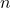is non-negative integer and it is called the degree of polynomial function. The valueis leading coefficient for variable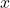with highest power, that is,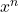. Finally, all coefficients are real numbers.

### What Is Not Polynomial Function ?

As we mentioned earlier, the coefficients can be any real number, however, the exponents value must be a non-negative integer.

Example #1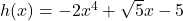The function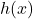is a polynomial of degree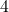because the variablehas non-negative integer exponent and the coefficients are real numbers. The negative numbers and radicals are also real numbers.

Example #2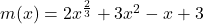The function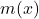is not a polynomial function because it has a fraction exponent, it must be a non-negative integer exponent.

Example #3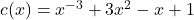The exponent in the leading term has a negative value, all exponent in polynomial must be greater than or equal to 0, meaning a non-negative integer.

### Polynomial Types Based on Number Of Terms

The terms of polynomial are separated by arithmetic operators such as plus (+) and minus (-). Based on number of terms a polynomial can be classified into:

• Monomial
• Binomial
• Trinomial

#### Monomial

A monomial is a single term polynomial. A single term can be a constant or a term with variable.

Example #4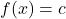where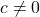.

Note that the variableis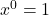therefore,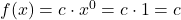, and it is also known as constant polynomial.

Example #5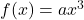where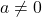.

#### Binomial

If the polynomial has only two terms, then it is known as a binomial.

Example #6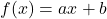where.

The above is example of binomial polynomial, but the degree is one. Such a polynomial is called linear function.

#### Trinomial

The trinomials have three terms.

Example #7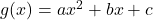whereThese type of polynomial are trinomials and a trinomial with degree two is called a quadratic equation. You can read previous article to know more about quadratic functions.

### Graph Of Polynomial Functions

The graph of polynomial function has two characteristic:

• The graph is continuous, meaning it has no breaks.
• The graph is smooth, meaning the graph is a single function with out sharp edge.

A continuous function can be a piecewise function which is not graph of polynomial.

You can recognize the graph of a polynomial just by looking at the smoothness and continuity. Any sharp corner in graph is not a polynomial function.

#### End Behavior of Polynomial Functions

If you notice that the graph of polynomial has two ends – rightmost end and leftmost end. The end behavior depends on what kind of polynomial we are dealing with. The graph may go up or down during intervals, but the ends behavior depends of a polynomial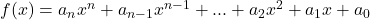depends on

• coefficientof the leading term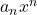in the polynomial.
• value of exponentin the leading term.

You can see from the figure 2, that when the value of variableincreases, only leading term dominates , all smaller terms are insignificant. Therefore, we perform a leading coefficient test to determine the end behavior of the polynomial function.

Whenis odd:

Whenis even:

The even polynomial has both ends pointing to same direction.

Figure 6 is graph of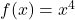and figure 7 is graph of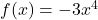.

Example #8

Determine the end behavior of the following function: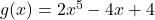.

Solution:

From the graph above it is easy to understand that this is an odd polynomial function with degree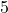. The leading coefficient is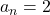which is greater than 0, that is,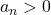.

Therefore, the graph of has leftmost end decreasing and rightmost end increasing as variableincreases without bound.

### Zeros Of Polynomial Function

If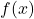is a polynomial function, then value offor which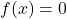is called zero of polynomials. There can be more than one zeros of a polynomial function.

The zeros of a polynomial function are called roots or solutions to the function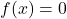. They appear as x-intercept in the graph of a polynomial function.

Example #8

Find the zeros of the polynomial: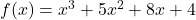.

Solution:

This problem cannot be solved with grouping technique. So checking whether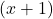is a solution.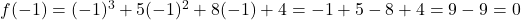Therefore,is a solution.

Usingwe can perform a polynomial division to find the roots.

\begin{aligned}
&\frac{x^3 +5x^2 + 8x + 4}{x + 1} = x^2 + 4x + 4 = (x + 2)^2
\end{aligned}

Therefore, the roots areand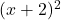.

#### Multiplicities of the Root

In the above example, the root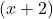is repeated two times and not crossing the x-axis The graph shows that rootwhich crosses the x-axis. This is called multiplicity of the root.

• If the multiplicity of the root is even, that means if the root repeat itself even times, it does not cross the x-axis.
• If the multiplicity of the root is odd, then the root cross the x-axis.

The reason why graph does not cross the x-axis when the multiplicity is even is because when the multiciplity is even, the sign of thedoes not change at all.

Example #9

Consider the following graph of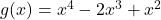which has two roots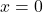and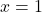. The factors of the function are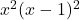.

The graph clearly shows that the function does not cross the the x-axis ator.

Example #10

In this example, we have a polynomial function :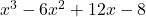whose factor is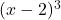. The x-intercept is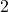.

The graph crosses the x-axis atbecause the multiplicity of the root is odd.